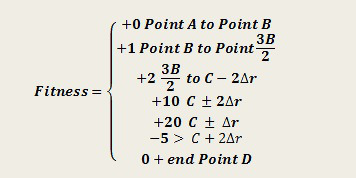# Self Learning Toy Helicopter

A cool project that uses a simple RC helicopter to demonstrate a neural netowrk based machine learning algorithm. For now its just a 1D algorithm, but the nice thing is being simple its easier to understand so you can use it with your next project if you want. You can read the full details here and a nice summary is coped below from hackaday

Consider the image above. The goal is for the helicopter to start at Point A, go to Point C and hover. Allotted time is 10 seconds per run. It has to teach itself how to do this and do it as quickly as possible. Remember, it knows where these points are via IR sensors. [Akshay] and [Sergio] developed an equation using a piecewise function to determine which runs were closest to Point C for the longest amount of time.Each of the points in the above equation is known via a voltage from the IR sensors, with Point A being 0.1 volts and Point D being 3.7 volts. The equation is designed to give the greatest value for the longest time spent at Point C. This value is known as a Fitness Value.

A neural network is used to determine at what level the throttle should be at to achieve the highest Fitness Value. This network is apart the Evolutionary Algorithm that runs in the firmware. Basically, it starts off with random values that generate random levels of throttle. The values that achieve the highest Fitness Value get ‘mutated’, while the others are discarded.

The mutations in the values are done at random, and the process repeats. In the end, the firmware learns the best throttle levels to achieve the goal of being at Point C for the longest time in the allotted 10 seconds.